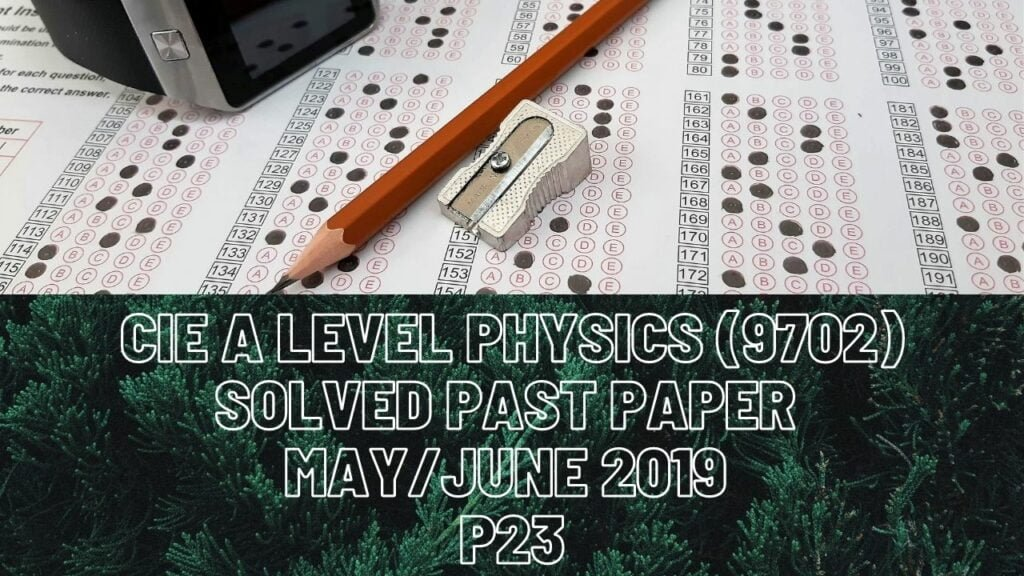A Level Physics### CIE A Level Physics Solved Past Paper May/June 2019 P23

2 bi) Distance is the actual path covered and displacement is the shortest route from starting point to the finishing point.

ii) According to law of conservation of energy, energy possessed by the ball at point X is going to be equal to that at point Y. At point X, ball only had kinetic energy of 4.5J and at point Y, it will have kinetic energy as well as gravitational potential energy since it covered a height h above the ground level.

iii) With an increase in h or the farther it travels away from the ground, speed starts to decrease and ultimately gets zero at the highest point it could get above the ground level.

3a) Rate of work done is power which is equal to (force*distance in the direction of force)/time taken).

4a) Field lines start from more positively charged plate to less positively charged plate

d) Time interval to reach from point X to point Y for both horizontal and vertical components is equal.

fi) When ratio charge/mass decreases, vertical motion of the particle has nothing to do with it because all quantities remain the same.

ii) Horizontal component of acceleration will decrease due to less charge of the particle

5b) At anti-nodes, amplitude is the highest and at nodes, amplitude gets zero.

ci) Vibration will be in the direction of propagation

ii) Phase difference between any 2 points exists only when there is at least one node between them.

e) When frequency increases, wavelength decreases and the next possible stationary wave forming in the tube with both its ends opened is with 2 nodes and 3 anti-nodes though length of the tube remains the same. In this new stationary wave form, one complete wavelength fits thus the new wavelength is 0.6m unlike previous wave form where only half wave could fit in the tube and the wavelength was of 1.2m. NOTE if the tube is opened at either sides, an anti-node must appear at that end and at closed end, node is supposed to appear.

6 ai) Current splits in parallel loop thus total current in the battery are current across resistor X and that across Y or Z (Y and Z are in series so they share the same current). The voltage across X and Y is equal to that across Z BUT voltage across X is not equal to the voltage across Y. Voltage across Z is 4.8V meaning some of it is for X and the rest is for Y. Y takes (2*1.8=3.6V) thus X is left with p.d of 1.2V and its resistance=1.2/1.8=0.67 ohms. Emf is equal to p.d across the loop and lost volts due to the battery’s internal resistance. We know that voltage across both loops is equal and that is 4.8V plus lost volts which are (1.5*2.4=3.6V) makeup emf and that’s 8.4V